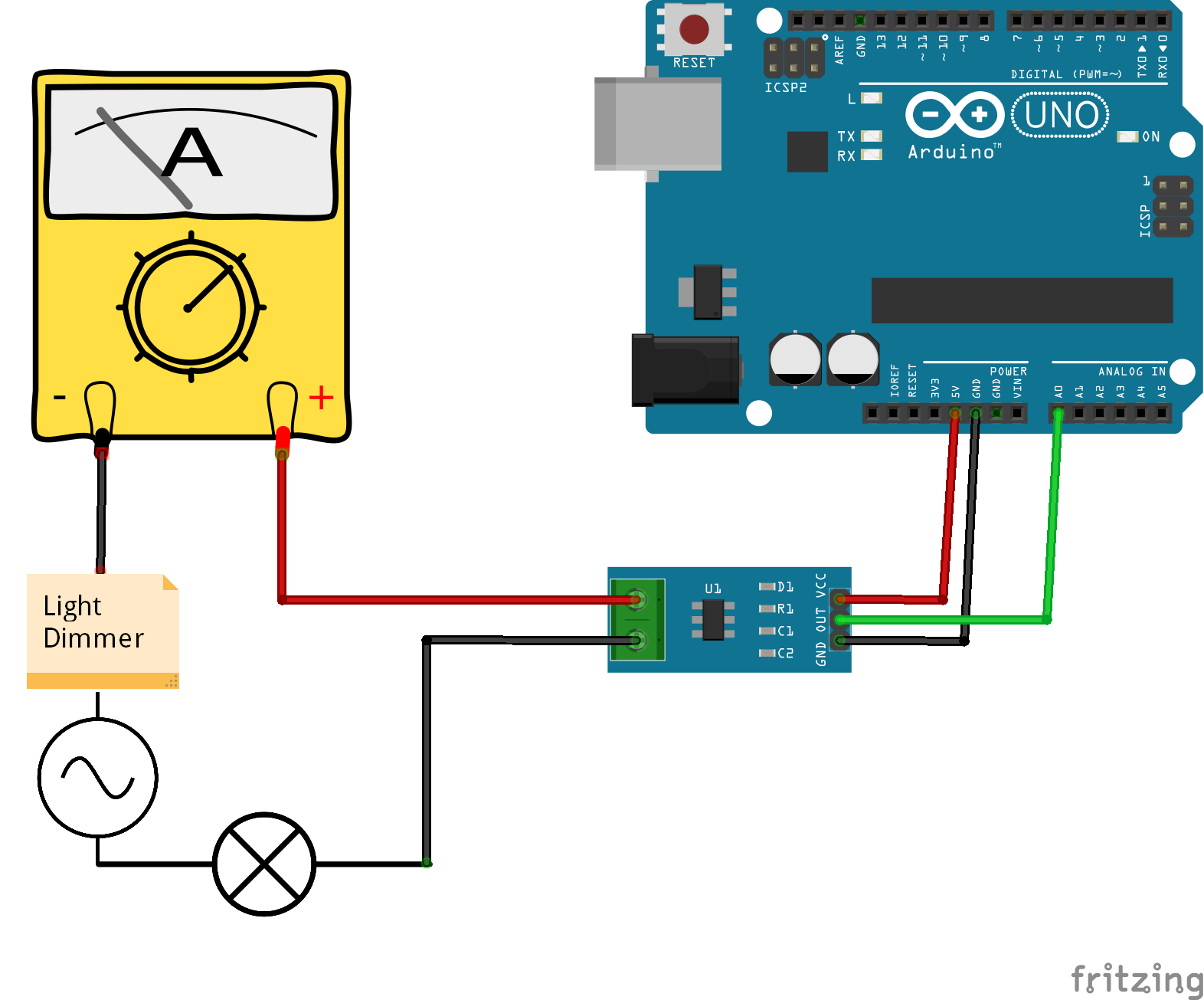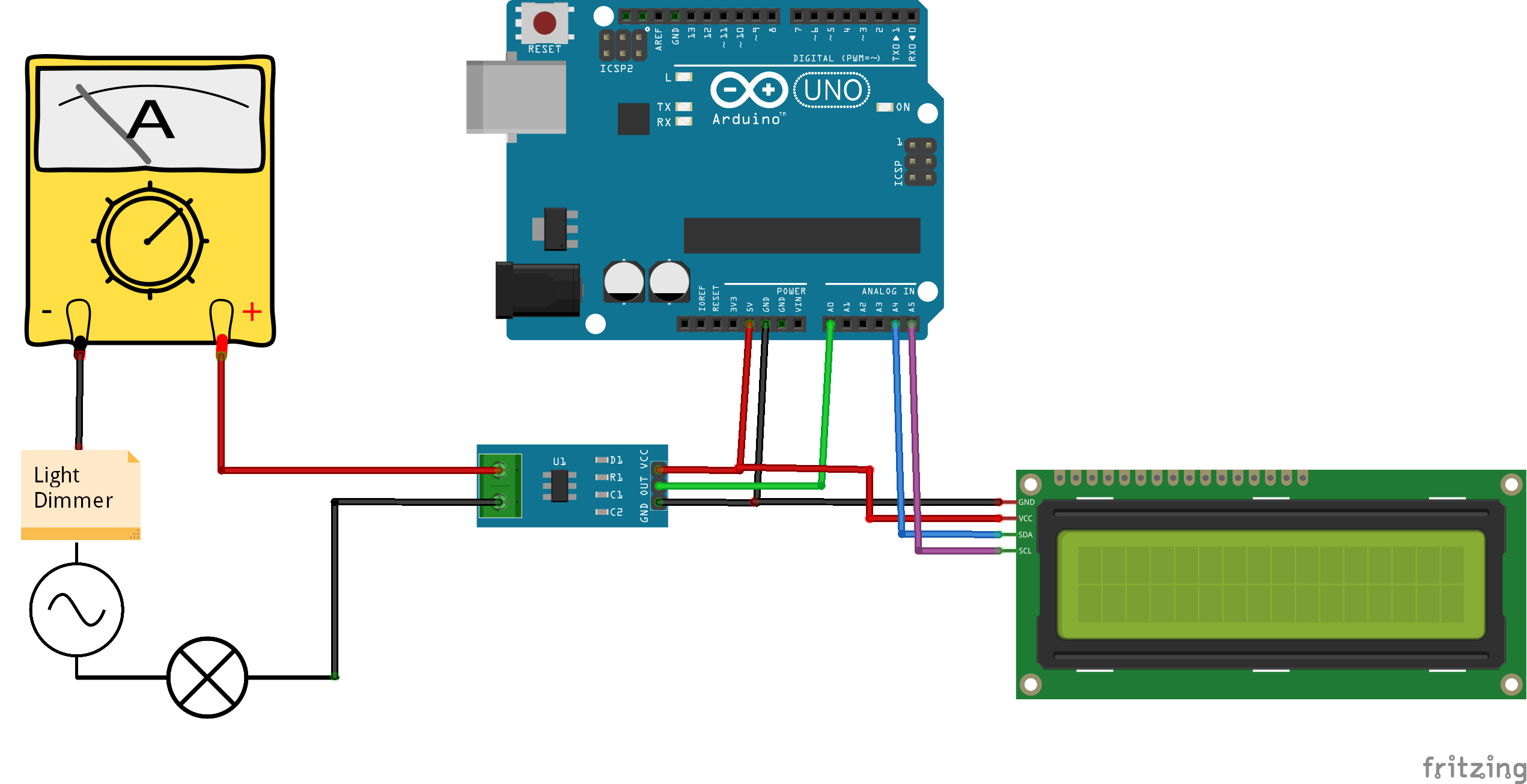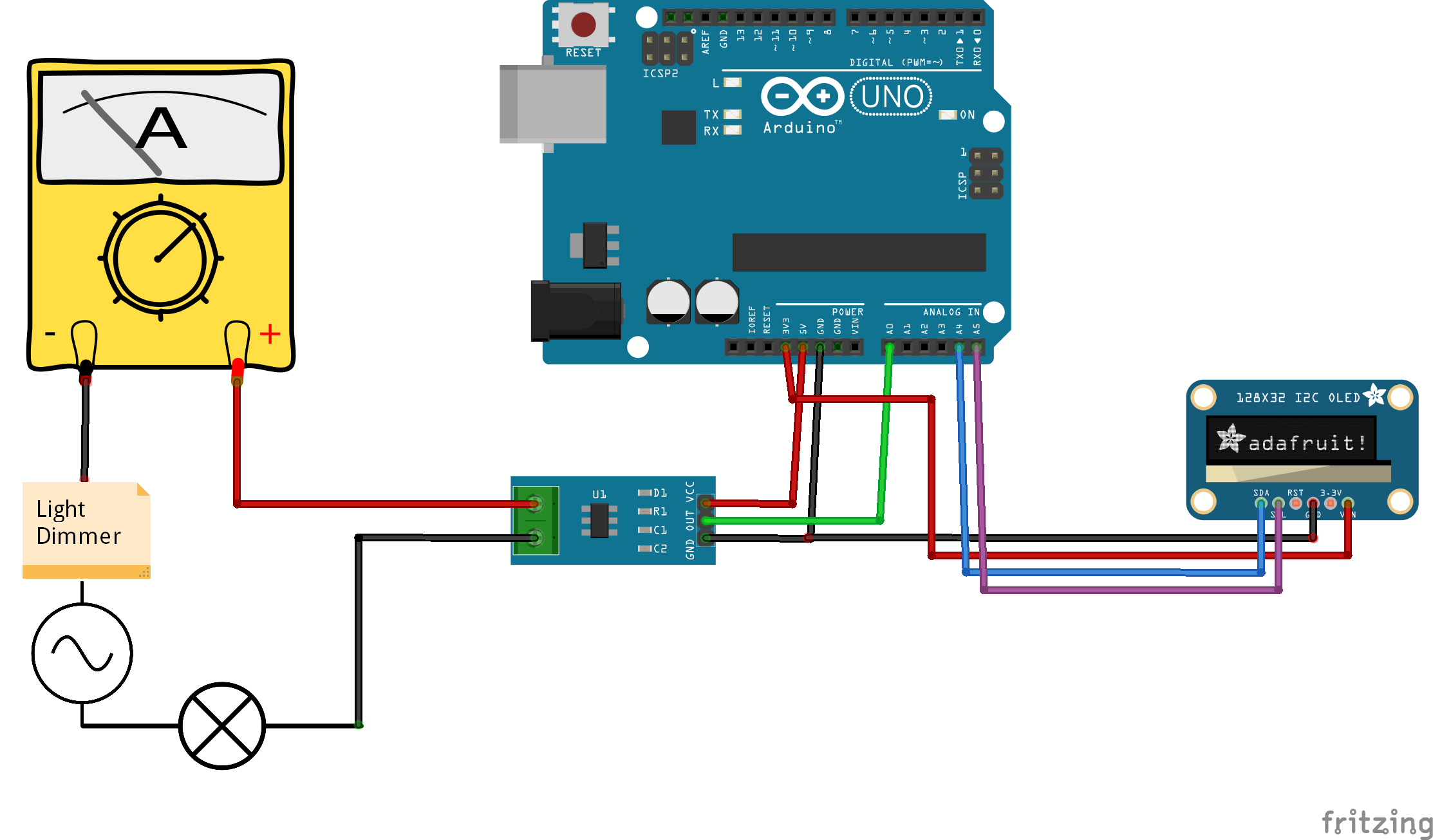Project tutorial# Measure Any AC Current with ACS712 © GPL3+

Module tutorial, signal visualisation and a simple code to measure not only sinewave signals but all types, like TRMS Ammeter. + LCD/OLED.

• 1,246 views
• 7 respects

## Components and suppliesArduino UNO & Genuino UNO
×1
 OLED i²c 128x32
×1
 ACS712 30A
×1
 LCD 16x2 i²c
×1

PLEASE BE CAREFUL IF YOU’RE USING THE POWER LINE

Hello, and welcome to another tutorial, this one is about measuring Alternating Current (AC), using ACS712 I’m using the 30Amps version which is ACS712 30A, and our lovely Arduino Uno board, I tried to add an OLED screen but unfortunately ended up breaking it while shooting the tutorial so I switched to the LCD, but below you’ll find the wiring and codes for both versions.

And by “Any AC…” I mean that we gonna see a code/library that works for all signal types not only sinewaves one, and our Ammeter will be able to calculate the TRUE ROOT MEAN SQUARE. And note that the sensor uses the Hall Effect (production of a voltage difference across an electrical conductor, transverse to an electric current in the conductor and to an applied magnetic field perpendicular to the current)

You can combine this project with this one: Easy measure of AC Voltage using Arduino and ZMPT101B

Tests will be done on an incandescent light bulb, controlled by a light dimmer in series with a reference multimeter and the ACS712 current sensor module.

Here’s the plan:

• First we need to interface our module with the Arduino board and check the signal shape when the dimmer is ON – Full Cycle – Half Cycle.
• Then we gonna check a simple code that doesn’t require any library, but it works only with Sinewaves signal (it will be tested).
• After that we gonna see the code that will measure the TRMS of the AC, and use the LCD.

### Parts

Please be carfeul when chosing the ACS712, don’t try to play it safe like I did (by purchasing a 30Amps version), this will just give big fluctuation when you try to use it for a domestic use or small amps applications, so here for me a 5A or 10A version would have been fine. Also note that 30A or 20A … will affect your calibrations too, but it’s explained how to calibrate in video and in codes comments.

Those are the parts I used, you can replace the UNO with any compatible board, or that LCD i²c with an OLED or any display you prefer.

### Test 1

Wiring and code

The wiring for test 1 can be found below (Wiring 1), alongside the code (Code 1).

Results

Upload the code and launch the serial plotter, you should be able to see a sinewave like signal, the red line shows when I turned down the dimmer and the current started having that shape that you usually find in a triac.

### Test 2

Now if you want to measure the current using the code above only, you’ll get values around 512 – 511, they don’t reflect the RMS value at all. So to measure the signal RMS value we gonna keep the same wiring as above but now we need another code that can calculate the RMS.

Wiring and code

The wiring is the same as (Wiring 1), but with a different code can be found below (Code 2).

Results

As you can see the values on the left (RMS) are the same as the value measured by the multimeter, on the right those are Peak to Peak values, and note that the dimmer is at full cycle as I’m using 75W bulb and 230VAC/50Hz.

!!!!!!!!!!!!!!!! BUT !!!!!!!!!!!!!!!!!!!!!

Here I turned all the way down my light dimmer, which means I’m approximately around 1/4 of a cycle, the multimeter is giving me 0.21A but my code gives me 0.36A, the code still measures from Peak to Peak, but as you can see above my signal is not linear when I turn the dimmer all the way down.

And that’s the difference between a TRMS measuring and a measuring that works only with sinewaves signal.

### Test 3

Now you see the problem of the code above, but fortunately there’s a library to the rescue, I really appreciate that library for all the work it does because as you know if you want to calculate a RMS value you need to use Integral Calculus, and that’s way difficult to achieve on an electronic development board, you can check the library cpp file to see what methods are used like the “Average” “Sigma”… Things related to statistics/probabilities ….

Wiring, code and library

The wiring is the same as wiring used above (Wiring 1), and below you can find the code (Code 3).

Results

The correct results are on the middle, the left ones are without calibration, and the right ones are used to demonstrate how to calibrate (check the video).

As you saw the huge difference between the two codes response to a non linear signal but the second one act as a TRMS multimeter.

### Test with LCD/OLED

Here we keep the same things all we do is instead of using the serial monitor we can use LCD or OLED

Wiring, codes and libraries

The wirings can be found below (Wiring_LCD) and (Wiring_OLED), codes also below (Code_ACS712_LCD) and (Code_ACS712_OLED).

Here are the OLED libraries

And here's the LCD_i2c_library

Result

This is the only test I could do because I broke my OLED screen while trying to shoot the video tutorial. Overall this works the same way as we saw before, it just displays it on a LCD i²c screen. (Yes the TRMS values not the peak-to-peak method).

That’s all folks, I hope you like this tutorial don’t forget to like the video tutorial and subscribe to SurtrTech channel if you want to show support, thank you very much.

Any question leave it in the comments.

## Code

##### Code_1.inoArduino
This code is to test the module and visualize the signal shap
```/*This code works with ACS712 Current sensor, it permits to read the raw data
It's better to use it with Serial Plotter
More details on www.surtrtech.com
*/

float i;

void setup() {

Serial.begin(9600);
pinMode(Current_sensor, INPUT);

}

void loop() {
Serial.println(i);
delay(100);                     //Modifying or removing the delay will change the way the signal is shown
//set it until you get the correct sinewave shap

}
```
##### Code_2.inoArduino
This code uses the peak-to-peak measuring method to calculate the RMS of a sinewave signal
```/* This code works with ACS712 current sensor, it permits to calculate the RMS of a sinewave Alternating Current
* it uses the Peak to Peak method to calculate the RMS
*/

#define SAMPLES 300   //Number of samples you want to take everytime you loop
#define ACS_Pin A0    //ACS712 data pin analong input

float High_peak,Low_peak;         //Variables to measure or calculate
float Amps_Peak_Peak, Amps_RMS;

void setup()
{
Serial.begin(9600);
pinMode(ACS_Pin,INPUT);        //Define pin mode
}

void loop()
{

Amps_RMS = Amps_Peak_Peak*0.3536*0.06;     //Now we have the peak to peak value normally the formula requires only multiplying times 0.3536
//but since the values will be very big you should multiply by 0.06, you can first not use it,
//do your calculations and compare them to real values measured by an Ammeter. eg: 0.06=Real value/Measured value

Serial.print(Amps_RMS);                    //Here I show the RMS value and the peak to peak value, you can print what you want and add the "A" symbol...
Serial.print("\t");
Serial.println(Amps_Peak_Peak);
delay(200);
}

void read_Amps()            //read_Amps function calculate the difference between the high peak and low peak
{                           //get peak to peak value
int cnt;            //Counter
High_peak = 0;      //We first assume that our high peak is equal to 0 and low peak is 1024, yes inverted
Low_peak = 1024;

for(cnt=0 ; cnt<SAMPLES ; cnt++)          //everytime a sample (module value) is taken it will go through test
{
float ACS_Value = analogRead(ACS_Pin); //We read a single value from the module

if(ACS_Value > High_peak)                //If that value is higher than the high peak (at first is 0)
{
High_peak = ACS_Value;            //The high peak will change from 0 to that value found
}

if(ACS_Value < Low_peak)                //If that value is lower than the low peak (at first is 1024)
{
Low_peak = ACS_Value;             //The low peak will change from 1024 to that value found
}
}                                        //We keep looping until we take all samples and at the end we will have the high/low peaks values

Amps_Peak_Peak = High_peak - Low_peak;      //Calculate the difference
}
```
##### Code_3.inoArduino
This code uses statistics formulas to calculate the TRMS of a signal whatever the sape and displays it on the serial monitor
```/* This code works with ACS712 current sensor, it permits the calculation of the signal TRMS
* Visit www.surtrtech.com for more details
*/

#include <Filters.h>                      //This library does a massive work check it's .cpp file

float ACS_Value;                              //Here we keep the raw data valuess
float testFrequency = 50;                    // test signal frequency (Hz)
float windowLength = 40.0/testFrequency;     // how long to average the signal, for statistist

float intercept = 0; // to be adjusted based on calibration testing
float slope = 0.0752; // to be adjusted based on calibration testing
//Please check the ACS712 Tutorial video by SurtrTech to see how to get them because it depends on your sensor, or look below

float Amps_TRMS; // estimated actual current in amps

unsigned long printPeriod = 1000; // in milliseconds
// Track time in milliseconds since last reading
unsigned long previousMillis = 0;

void setup() {
Serial.begin( 9600 );    // Start the serial port
pinMode(ACS_Pin,INPUT);  //Define the pin mode
}

void loop() {
RunningStatistics inputStats;                 // create statistics to look at the raw test signal
inputStats.setWindowSecs( windowLength );     //Set the window length

while( true ) {
inputStats.input(ACS_Value);  // log to Stats function

if((unsigned long)(millis() - previousMillis) >= printPeriod) { //every second we do the calculation
previousMillis = millis();   // update time

Amps_TRMS = intercept + slope * inputStats.sigma();

Serial.print( "\t Amps: " );
Serial.print( Amps_TRMS );

}
}
}

/* About the slope and intercept
* First you need to know that all the TRMS calucations are done by functions from the library, it's the "inputStats.sigma()" value
* At first you can display that "inputStats.sigma()" as your TRMS value, then try to measure using it when the input is 0.00A
* If the measured value is 0 like I got you can keep the intercept as 0, otherwise you'll need to add or substract to make that value equal to 0
* In other words " remove the offset"
* Then turn on the power to a known value, for example use a bulb or a led that ou know its power and you already know your voltage, so a little math you'll get the theoritical amps
* you divide that theory value by the measured value and here you got the slope, now place them or modify them
*/
```
##### Code_ACS712_LCD.inoArduino
This code uses the TRMS calculation method and displays it on the LCD i²c
```/* This code works with ACS712 and LCD ic
* It measure the TRMS of an Alternating Current and displays the value on the screen
* Visit www.SurtrTech.com for more details
*/

#include <Filters.h>              //This library does a huge work check its .cpp file
#include <LiquidCrystal_I2C.h>    //LCD ic library

#define ACS_Pin A0              //ACS712 data pin

#define I2C_ADDR 0x27 //I2C adress, you should use the code to scan the adress first (0x27) here
#define BACKLIGHT_PIN 3 // Declaring LCD Pins
#define En_pin 2
#define Rw_pin 1
#define Rs_pin 0
#define D4_pin 4
#define D5_pin 5
#define D6_pin 6
#define D7_pin 7

float testFrequency = 50;                     // test signal frequency (Hz)
float windowLength = 40.0/testFrequency;     // how long to average the signal, for statistist

float intercept = 0; // to be adjusted based on calibration testing
float slope = 0.0752; // to be adjusted based on calibration testing
//Please check the ACS712 Tutorial video by SurtrTech to see how to get them because it depends on your sensor, or look below

float Amps_TRMS;
float ACS_Value;

unsigned long printPeriod = 1000;
unsigned long previousMillis = 0;

void setup() {
digitalWrite(2,HIGH);
lcd.begin (16,2);
lcd.setBacklightPin(BACKLIGHT_PIN,POSITIVE);
lcd.setBacklight(HIGH); //Lighting backlight
lcd.home ();

}

void loop() {
RunningStatistics inputStats;                 // create statistics to look at the raw test signal
inputStats.setWindowSecs( windowLength );

while( true ) {
inputStats.input(ACS_Value);  // log to Stats function

if((unsigned long)(millis() - previousMillis) >= printPeriod) { //every second we do the calculation
previousMillis = millis();   // update time

Amps_TRMS = intercept + slope * inputStats.sigma();  //Calibrate the values
lcd.clear();               //clear the lcd and print in a certain position
lcd.setCursor(2,0);
lcd.print(Amps_TRMS);
lcd.print(" A");

}
}
}

/* About the slope and intercept
* First you need to know that all the TRMS calucations are done by functions from the library, it's the "inputStats.sigma()" value
* At first you can display that "inputStats.sigma()" as your TRMS value, then try to measure using it when the input is 0.00A
* If the measured value is 0 like I got you can keep the intercept as 0, otherwise you'll need to add or substract to make that value equal to 0
* In other words " remove the offset"
* Then turn on the power to a known value, for example use a bulb or a led that ou know its power and you already know your voltage, so a little math you'll get the theoritical amps
* you divide that theory value by the measured value and here you got the slope, now place them or modify them
*/
```
##### Code_ACS712_OLED.inoArduino
This code uses the TRMS calculation method and displays the value on the OLED.
```/* This code works with ACS712 and OLED ic
* It measure the TRMS of an Alternating Current and displays the value on the screen
* Visit www.SurtrTech.com for more details
*/

#include <Filters.h>            //This library does a huge work check its .cpp file

#define ACS_Pin A0

#define SCREEN_WIDTH 128 // OLED display width, in pixels
#define SCREEN_HEIGHT 32 // OLED display height, in pixels
#define OLED_RESET    -1 // Reset pin # (or -1 if sharing Arduino reset pin)

float testFrequency = 50;                     // test signal frequency (Hz)
float windowLength = 40.0/testFrequency;     // how long to average the signal, for statistist

float intercept = 0; // to be adjusted based on calibration testing
float slope = 0.0752; // to be adjusted based on calibration testing
//Please check the ACS712 Tutorial video by SurtrTech to see how to get them because it depends on your sensor, or look below

float Amps_TRMS;
float ACS_Value;

unsigned long printPeriod = 1000;
unsigned long previousMillis = 0;

Adafruit_SSD1306 display(SCREEN_WIDTH, SCREEN_HEIGHT, &Wire, OLED_RESET); //Declaring the display name (display)

void setup() {
display.begin(SSD1306_SWITCHCAPVCC, 0x3C); //Start the OLED display
display.clearDisplay();
display.display();
}

void loop() {
RunningStatistics inputStats;                 // create statistics to look at the raw test signal
inputStats.setWindowSecs( windowLength );

while( true ) {
inputStats.input(ACS_Value);  // log to Stats function

if((unsigned long)(millis() - previousMillis) >= printPeriod) { //Do the calculations every 1s
previousMillis = millis();   // update time

Amps_TRMS = intercept + slope * inputStats.sigma();
display.clearDisplay();
display.setTextSize(3);
display.setTextColor(WHITE);
display.setCursor(15,10);
display.print(Amps_TRMS);
display.println(" A");
display.display();
}
}
}

/* About the slope and intercept
* First you need to know that all the TRMS calucations are done by functions from the library, it's the "inputStats.sigma()" value
* At first you can display that "inputStats.sigma()" as your TRMS value, then try to measure using it when the input is 0.00A
* If the measured value is 0 like I got you can keep the intercept as 0, otherwise you'll need to add or substract to make that value equal to 0
* In other words " remove the offset"
* Then turn on the power to a known value, for example use a bulb or a led that ou know its power and you already know your voltage, so a little math you'll get the theoritical amps
* you divide that theory value by the measured value and here you got the slope, now place them or modify them
*/
```

## Schematics

Wiring used of tests 1,2 and 3Use LCD i²cUse OLED 128x32#### Published on

December 12, 2019

#### Members who respect this project

See similar projects
you might like

• 4,648 views
• 9 respects

#### Measure Any AC Voltage Up to 250V

Project tutorial by SurtrTech

• 15,585 views
• 10 respects

#### Measure Heart Rate and SpO2 with MAX30102

Project tutorial by SurtrTech

• 52,234 views
• 158 respects

#### BMP280: Measure Temperature, Pressure and Altitude

Project tutorial by SurtrTech

• 19,280 views
• 46 respects

#### ToF 10120 Laser Rangefinder to Measure Distance + LCD

Project tutorial by SurtrTech

• 13,147 views